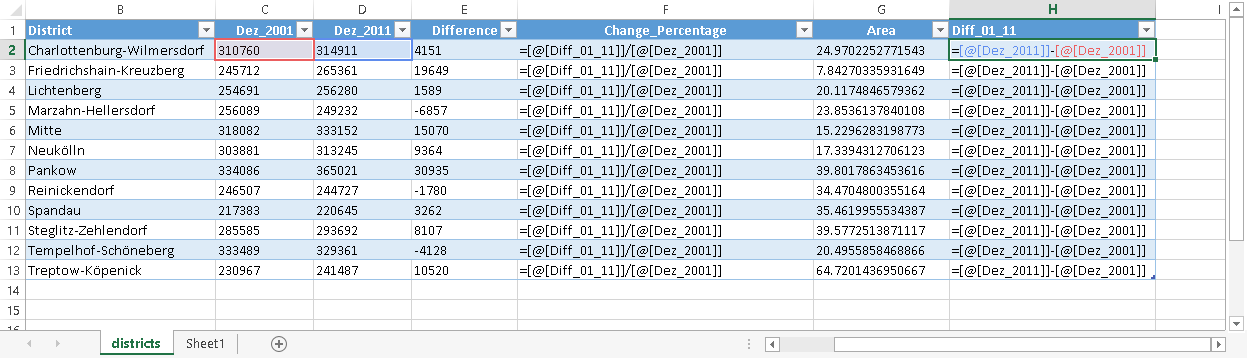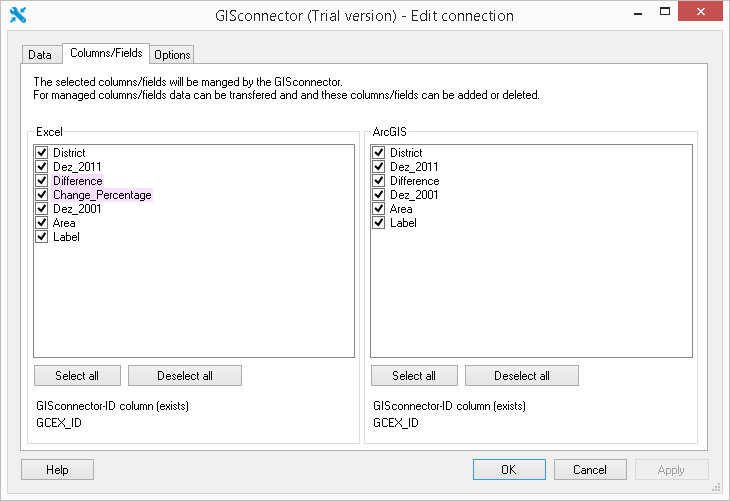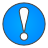To properly display this page you need a browser with JavaScript support.GISconnector for Excel Help
Index
 Getting started Excel formulas and the GISconnector

# Excel formulas and the GISconnector

The central function of Excel – in contrast to that of a database – is in its capacity to make use of formulas that can be entered into cells as a dynamic equation. For this reason, we have given particular consideration to the concept of “Formulas” in the development of the GISconnector for Excel. It is important to understand how the GISconnector for Excel operates with formulas.
Check out our video channel with demos and tutorials at Vimeo.

Prerequisite
A connection between an ArcGIS feature-class or table and an Excel table must be available.
If you have not yet applied a connection, you have the following three possibilities for doing so:

The same workflows to establish a connection are available from
the Excel side, too:

You can see below a part from an Excel table for which a connection to a feature-class exists. The option "Show formulas" has been activated under the index "Formulas". In column F (Change_Percentage) and H (Difference) two formulas can be seen.The GISconnector for Excel recognises if a formula has been entered for a column. In the dialogue Edit connection under the index Columns/fields you can see in which column a formula has been typed.
The column concerned, in this case "Change_Percentage" and “Difference”, are marked in pink. In the case given, the column “Change_Percentage” does not exist in ArcGIS yet.To what extent does a formula in an Excel column influence the behavior of the GISconnector for Excel?

We assume that a formula in Excel is used in order to dynamically calculate a specific result. For this reason it is not possible for a column with formulas to be overwritten with values by means of the data transfer from ArcGIS to Excel. If the relevant column in the Columns/fields index of the Edit connection dialogue is managed on the ArcGIS side by the GISconnector for Excel, this has no effect in a case whwhen a formula has been assigned to this column in Excel. Under no circumstances is the formula overwritten when transferring from ArcGIS to Excel.

However, if you would like to overwrite the column assigned a formula with values from ArcGIS, copy the column with the formula into the clipboard and then insert it again using the option “Insert values". Now the column in Excel no longer contains a formula, having only the last values that were calculated. The column concerned is now under the Columns/fields index of the Edit connection dialogue. It is no longer marked in pink and can at any time be overwritten with values from ArcGIS.Important Notice:
Columns with formulas can be deleted when transferring from ArcGIS to Excel. Read about this further in the chapter "Deleting rows and columns".